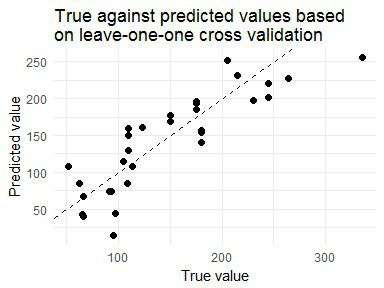# Easy leave-one-out cross validation with pipelearner

@drsimonj here to show you how to do leave-one-out cross validation using pipelearner.

## Leave-one-out cross validation #

Leave-one-out is a type of cross validation whereby the following is done for each observation in the data:

• Run model on all other observations
• Use model to predict value for observation

This means that a model is fitted, and a predicted is made n times where n is the number of observations in your data.

## Leave-one-out in pipelearner #

pipelearner is a package for streamlining machine learning pipelines, including cross validation. If you’re new to it, check out blogR for other relevant posts.

To demonstrate, let’s use regression to predict horsepower (`hp`) with all other variables in the `mtcars` data set. Set this up in pipelearner as follows:

``````library(pipelearner)

pl <- pipelearner(mtcars, lm, hp ~ .)
``````

How cross validation is done is handled by `learn_cvpairs()`. For leave-one-out, specify k = number of rows:

``````pl <- learn_cvpairs(pl, k = nrow(mtcars))
``````

Finally, `learn()` the model on all folds:

``````pl <- learn(pl)
``````

This can all be written in a pipeline:

``````pl <- pipelearner(mtcars, lm, hp ~ .) %>%
learn_cvpairs(k = nrow(mtcars)) %>%
learn()

pl
#> # A tibble: 32 × 9
#>    models.id cv_pairs.id train_p      fit target model     params
#>        <chr>       <chr>   <dbl>   <list>  <chr> <chr>     <list>
#> 1          1          01       1 <S3: lm>     hp    lm <list >
#> 2          1          02       1 <S3: lm>     hp    lm <list >
#> 3          1          03       1 <S3: lm>     hp    lm <list >
#> 4          1          04       1 <S3: lm>     hp    lm <list >
#> 5          1          05       1 <S3: lm>     hp    lm <list >
#> 6          1          06       1 <S3: lm>     hp    lm <list >
#> 7          1          07       1 <S3: lm>     hp    lm <list >
#> 8          1          08       1 <S3: lm>     hp    lm <list >
#> 9          1          09       1 <S3: lm>     hp    lm <list >
#> 10         1          10       1 <S3: lm>     hp    lm <list >
#> # ... with 22 more rows, and 2 more variables: train <list>, test <list>
``````

## Evaluating performance #

Performance can be evaluated in many ways depending on your model. We will calculate R2:

``````library(tidyverse)

# Extract true and predicted values of hp for each observation
pl <- pl %>%
mutate(true = map2_dbl(test, target, ~as.data.frame(.x)[[.y]]),
predicted = map2_dbl(fit, test, predict))

# Summarise results
results <- pl %>%
summarise(
sse = sum((predicted - true)^2),
sst = sum(true^2)
) %>%
mutate(r_squared = 1 - sse / sst)

results
#> # A tibble: 1 × 3
#>        sse    sst r_squared
#>      <dbl>  <dbl>     <dbl>
#> 1 41145.56 834278 0.9506812
``````

Using leave-one-out cross validation, the regression model obtains an R2 of 0.95 when generalizing to predict horsepower in new data.

We’ll conclude with a plot of each true data point and it’s predicted value:

``````pl %>%
ggplot(aes(true, predicted)) +
geom_point(size = 2) +
geom_abline(intercept = 0, slope = 1, linetype = 2)  +
theme_minimal() +
labs(x = "True value", y = "Predicted value") +
ggtitle("True against predicted values based\non leave-one-one cross validation")
``````## Sign off #

Thanks for reading and I hope this was useful for you.

For updates of recent blog posts, follow @drsimonj on Twitter, or email me at drsimonjackson@gmail.com to get in touch.

If you’d like the code that produced this blog, check out the blogR GitHub repository.